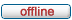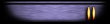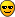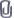bckobayashimaru.de

Bridge Commander Kobayashi Maru Project
 It is currently Tue Jun 25, 2019 4:18 am

 All times are UTC

 Print view Previous topic | Next topic
Author Message
 Post subject: Change in the calculation of the warpspeedPosted: Sat Jan 11, 2014 8:03 amCadet 2nd YearJoined: Fri Jan 03, 2014 10:33 pm
Posts: 30
Location: Eisenach, Germany
I changed the formula for calculating the warp speed based on the warp factors.
I've found a system behind the values years ago.

Here the values ​​from the Star Trek Encyclopedia:

Code:
Factor   -   Warpspeed
1.000   -   1
2.000   -   10
3.000   -   39
4.000   -   102
5.000   -   213
6.000   -   392
7.000   -   656
8.000   -   1024
9.000   -   1516
9.200   -   1649
9.600   -   1909
9.900   -   3053
9.990   -   7912
9.9999   -   199516

The values ​​correspond to an exponential function.

Between 1 and 9, the exponent value equal to the constant 3.3333

Code:
1 = 1^3.3333
10 = 2^3.333
39 = 3^3.3333
102 = 4^3.3333
213 = 5^3.3333
392 = 6^3.3333
656 = 7^3.3333
1024 = 8^^3.3333
1516 = 9^3.3333

About Warp 9 increases the exponent and tends to infinity as soon as the factor, the 10 approaches. Many people have now come up with any formulas that approximate this behavior. If you look at the exponents but even more closely, you can see a system behind it.

Code:
3053 = 9.9 ^3.5
7912 = 9.99 ^3.9
199516 = 9.9999 ^5.3

The numbers to be said not yet so much. But if you subtract 3.3 from the exponent in each case, a system is apparent.

Code:
3.5 - 3.3 = 0.2
3.9 - 3.3 = 0.6
5.3 - 3.3 = 2.0

A good mathematician sees the system now. For the less talented people I multiply these values ​​again with 5.

Code:
0.2 * 5 = 1
0.6 * 5 = 3
2.0 * 5 = 10

What shall we say to these values​​?

If we compared these values ​​to the number of 9s in the decimal of the warp factors.

Code:
9.9 -> 1 - 1
9.99 -> 2 - 3
9.9999 -> 4 - 10

This corresponds exactly to the number series of the partial sums of natural numbers.

Code:
1 - 1
2 - 1+2 = 3
3 - 1+2+3 = 6
4 - 1+2+3+4 = 10How to mathematically describe now the number of 9s in the decimal places?

Since it uses a simple "tricks".
If the warp factors of 10 is subtracted:

Code:
10-9.9 = 0.1
10-9.99 = 0.01
10-9.9999 = 0.0001

And from these figures the logarithm to the base 10 takes ...

Code:
log10(0.1) = -1
log10(0.01) = -2
Log(0.0001) = -4

Mathematics can sometimes be so elegand and easy.The partial sums calculated as follows: fPart = (n ^ 2 - n) / 2

fAux = -log10(10 - fWarpfactor)

-> fPart = (fAux^2 + fAux) / 2

But since I have these values ​​obtained by the multiplication by 5, I have to divide by 5, the result again.

fExp = (fAux^2 + fAux) / 10

And finally, of course, the 3.3 should add again.

fExp = (fAux^2 + fAux) / 10 + 3.3

fSpeed = fWarpfactor ^ fExp

This formula reproduces all canonical warp speeds for warp factors including about 9.9.

Between 9 and 9.9 there is not such a simple formula unfortunately. The reason for this is that the exponent of the warp factors were chosen as simple as possible by the authors of the encyclopedia. Then there is no mathematical correlation to these values​​.
Code:
1516 = 9.0 ^ 3.3333
1649 = 9.2 ^ 3.3381
1909 = 9.6 ^ 3.34
3053 = 9.9 ^ 3.50

The value for warp factor 9.6 is too small. In a clean model by mathematical exponent course of the exponent would be slightly larger. The exponent for 9.2 do however understand, but with a rather complicated formula. For the reason I have made it easy. The exponents for 9 and 9.9 we have already determined by suitable formulas that apply in each range of values.

If we look again the formula for the fractional part of the warp factors consider: fAux = log10(10 - fWarpfactor)
For 9.0, this formula yields 0, for 9.9, this formula gives a value of 1.

A value of 9, the exponent is 3.3333, at 9.9, the exponent should be 3.5. So I've tried from the gut simply following times:

fExp = 3.3333 * (1 - fAux) + 3.5 * fAux.

In 9 of the rear part is 0 and there is only (1-0) * 3.3333 left. This fits that way. At 9.9 the front part is 0 and there is only the rear 1 * 3.5 left. So this fits, too. Since both parts of the formula, a linear gradient of the same increase, the result for the intermediate values ​​is a continuous course of 3.3333 to 3.5.

So I hope I do not have now killed the reader with too much mathematics.Attachments:LibWarp.py [19.52 KiB] Downloaded 310 timesNormalWarp.py [27.65 KiB] Downloaded 288 timesEnhancedWarp.py [27.67 KiB] Downloaded 294 times
TopPost subject: Re: Change in the calculation of the warpspeedPosted: Wed Jan 22, 2014 12:17 amCadet 2nd YearJoined: Fri Jan 03, 2014 10:33 pm
Posts: 30
Location: Eisenach, Germany
Update: Repaired LibWarp-File (I forget "import math").

 Attachments:LibWarp.py [19.53 KiB] Downloaded 283 times
TopDisplay posts from previous: All posts1 day7 days2 weeks1 month3 months6 months1 year Sort by AuthorPost timeSubject AscendingDescending

 All times are UTC

Who is online

Users browsing this forum: No registered users and 1 guest

 You cannot post new topics in this forumYou cannot reply to topics in this forumYou cannot edit your posts in this forumYou cannot delete your posts in this forumYou cannot post attachments in this forum

Search for:
 Jump to:  Select a forum ------------------ Website    Website - General    Website - Misc    Website - Wiki BC: Kobayashi Maru    Kobayashi Maru - General    Kobayashi Maru - Support    Kobayashi Maru - Bugs    Kobayashi Maru - Feedback Bridge Commander    Bridge Commander - General    Bridge Commander - Modding    Bridge Commander - Scripting    Bridge Commander - Screenshots    Bridge Commander - Videos